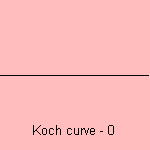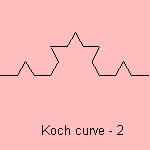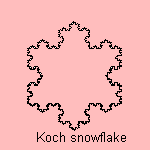# Koch curve

## fractal

 The Swedish mathematicion Niels Fabian Helge von Koch (1870-1924) constructed the Koch curve in 1904 as an example of a continuous, non-differentiable curve. Karl Weierstrass had demonstrated the existence of such a curve in 1872. Other names for the curve are Koch star and Koch island. The curve is a base motif fractal which uses a line segment as base. The motif is to divide the line segment into three equal parts and replace the middle by the two other sides of an equilateral triangle:The fractal dimension of the Koch curve is equal to log4/log3, what is about 1.2619 1). Three copies of the Koch curve placed at the the sides of an equilateral triangle, form a Koch snowflake:notes 1) Fractal dimension = log N / log e, where N is the number of line segments and e the magnification. For the Koch curve: N=4, e=3.